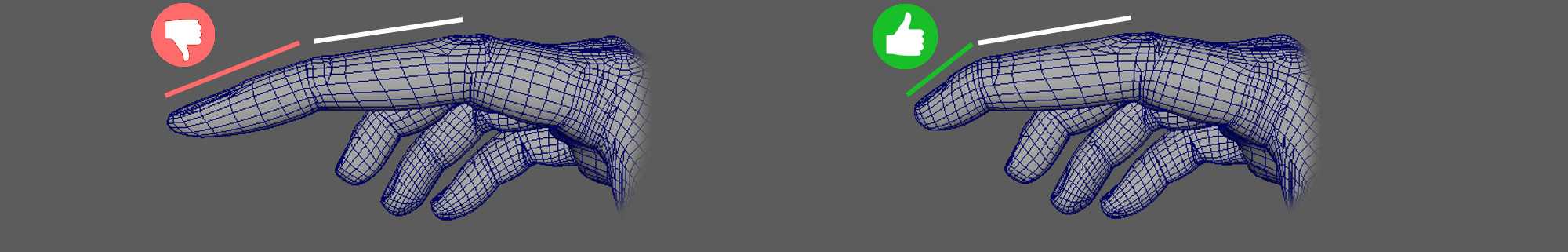# Dual Quaternion Skinning with scaleDQS with scale applied on the second to last joint. Left, globally propagates until the last bone, right, scale localized to each joint.

Here I'm merely paraphrasing Kavan's DQS article using my own notations and words. You should read my tutorial on Dual Quaternion Skinning (DQS) first. Once successfully implemented you can easily extend it to support scale given the following instructions. I will use the notations I introduced in my skeletal animation cheat sheet.

Unlike matrices, by definition Dual Quaternions are not intended to represent scale transformations. A dual Quaternion only represents a translation and a rotation. To represent all three transformations you must divide the skinning algorithm into two stages, first scale, then rotation and translation.

First you must apply Linear Blending Skinning (LBS) but only considering the scale component $$S_j$$ of the joints. This will have the effect of bulging your character in the rest pose. In the second stage you will apply DQS with the remaining rigid transformation $$R_j$$ and rotate the inflated skin.

There is absolutely no difficulties separating your skinning algorithm into those two stages. The difficult part is to compute the global scale matrix $$S_j$$ and computing the rigid matrix $$R_j$$ containing rotation and translation. $$S_j$$ is used in lieu of the LBS matrix $$T_j$$ and blended by the unaltered LBS routine. $$R_j$$ is converted to Dual Quaternion and blended through the unmodified DQS routine.

## Global propagation

You can allow the scale to propagate throughout the skeleton, any joint scale will also scale its children (top left figure). The advantage of this version is that you can directly use $$T_j$$.

By applying a polar decomposition on $$T_j$$ it will separate the transformation into two matrices, a rigid component and a scale component $$T_j = R_j S_j$$. I provide here polar decomposition routines for 3x3 matrices (you could also use Eigen).

std::vector<Mat4x4> scale(nb_bones);
std::vector<Dual_quat> rigid(nb_bones);
// Kynematic
for(int i = 0; i < nb_bones; ++i)
{
Mat4x4 tr = skinning_transformations[i]; // T_j
Polar_decomposition decomp( tr.get_mat3() );
scale[i] = Mat4x4(decomp.matrix3x3_S());
rigid[i] = Dual_quat(Mat4x4(decomp.matrix3x3_R(), tr.get_translation()));
}
// Deformation
for(int i = 0; i < nb_vertices; ++i)
{
Point3 in_vert = in_vertex_buffer[i]; // p_i
Vec3 in_normal = in_normal_buffer[i]; // n_i
Point3 out_vert;
Vec3 out_normal;
{
// p'i =  (sum over every influencing bone j)(w_ij T_j) p_i
// n'i = ((sum over every influencing bone j)(w_ij T_j))^(-1)^(T) n_i
out_vert = linear_blending(bone_weights[i], scale, in_vert, in_normal, out_normal);
// Apply DQS on top of the inflated mesh:
Dual_quat dq_blend = dual_quaternion_blending( bone_weights[i], rigid);
out_normal = dq_blend.rotate( out_normal );
out_vert   = dq_blend.transform(out_vert);
}
out_vertex_buffer[i] = out_vert; // p'i
out_normal_buffer[i] = out_normal.normalized(); // n'i
}


## Local scale

Localizing the scale only on specific joint of the skeleton is a little more tricky. The computation of $$R_j$$ and $$S_j$$ is more involved and you need access to the user local transformations $$Ul_j = Ur_j Us_j$$ at each joint. We assume access to $$Ur_j$$ and $$Us_j$$ but they can be obtain through polar decomposition.

$$\begin{equation*} \begin{split} S_j & = Ws_j (B_j)^{-1} \\ Ws_j & = Ls_{\text{root}} \ \cdots \ Ls_{p(j)} Ls_j Us_j \\ Ls_j & = \text{mat4}(rot(L_j), Us_{p(j)} \ \ trans(L_j) )\\ \end{split} \end{equation*}$$

• $$B_j$$ global bind matrix
• $$L_j$$ local bind matrix
• $$rot: \mathbb{R}^{4\times4} \to \mathbb{R}^{3\times3}$$ extract rotational part of a matrix
• $$trans: \mathbb{R}^{4\times4} \to \mathbb{R}^{3}$$ extract translational part of a matrix

$$\begin{equation*} \begin{split} R_j & = Wr_j (Ws_j Us_j^{-1})^{-1} \\ Wr_j & = Ls_{\text{root}} Ur_{\text{root}} \ \cdots \ Ls_{p(j)} Ur_{p(j)} Ls_j Ur_j \end{split} \end{equation*}$$

Then you only have to adapt the kinematic part of your code